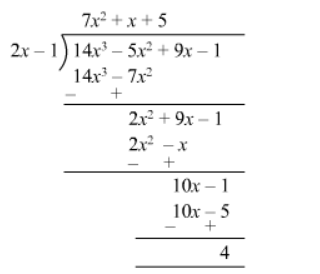# Divide the following and find the quotient and remainder.`
Question:

Divide 14x3 − 5x2 + 9x − 1 by 2x − 1 and find the quotient and remainder

Solution:Quotient $=7 x^{2}+x+5$

Remainder $=4$